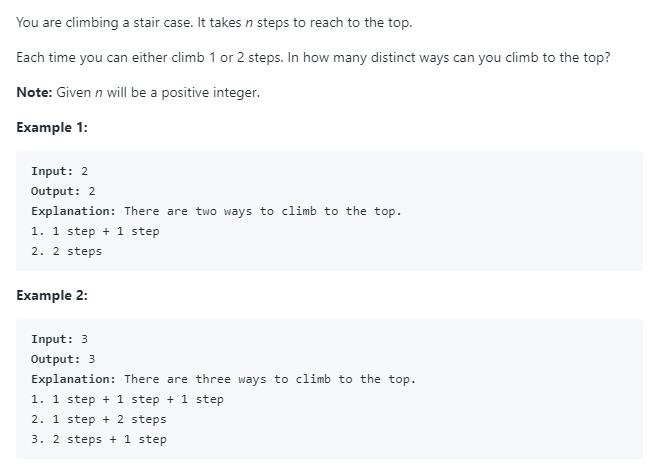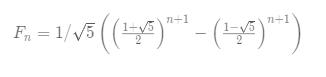# 题目描述（简单难度）# 解法一 暴力解法

f ( n ) = f ( n - 1) + f ( n - 2 )。

f ( 1 ) = 1，f ( 2 ) = 2 。

public int climbStairs(int n) {
return climbStairsN(n);
}

private int climbStairsN(int n) {
if (n == 1) {
return 1;
}
if (n == 2) {
return 2;
}
return climbStairsN(n - 1) + climbStairsN(n - 2);
}


# 解法二 暴力解法优化

public int climbStairs(int n) {
return climbStairsN(n, new HashMap<Integer, Integer>());
}

private int climbStairsN(int n, HashMap<Integer, Integer> hashMap) {
if (n == 1) {
return 1;
}
if (n == 2) {
return 2;
}
int n1 = 0;
if (!hashMap.containsKey(n - 1)) {
n1 = climbStairsN(n - 1, hashMap);
hashMap.put(n - 1, n1);
} else {
n1 = hashMap.get(n - 1);
}
int n2 = 0;
if (!hashMap.containsKey(n - 2)) {
n2 = climbStairsN(n - 2, hashMap);
hashMap.put(n - 2, n1);
} else {
n2 = hashMap.get(n - 2);
}
return n1 + n2;
}


public int climbStairs(int n) {
int memo[] = new int[n + 1];
return climbStairsN(n, memo);
}
private int climbStairsN(int n, int[] memo) {
if (n == 1) {
return 1;
}
if (n == 2) {
return 2;
}
int n1 = 0;
//数组的默认值是 0
if (memo[n - 1] == 0) {
n1 = climbStairsN(n - 1, memo);
memo[n - 1] = n1;
} else {
n1 = memo[n - 1];
}
int n2 = 0;
if (memo[n - 2] == 0) {
n2 = climbStairsN(n - 2, memo);
memo[n - 2] = n2;

} else {
n2 = memo[n - 2];
}
return n1 + n2;
}


# 解法三 迭代

public int climbStairs(int n) {
int n1 = 1;
int n2 = 2;
if (n == 1) {
return n1;
}
if (n == 2) {
return n2;
}
//n1、n2 都后移一个位置
for (int i = 3; i <= n; i++) {
int temp = n2;
n2 = n1 + n2;
n1 = temp;
}
return n2;
}


# 解法四 矩阵相乘

Solution5叫做 Binets Method，它利用数学归纳法证明了一下，这里就直接用了，至于怎么想出来的，我也不清楚了。

public int climbStairs(int n) {
int[][] Q = {{1, 1}, {1, 0}};
int[][] res = pow(Q, n);
return res;
}
public int[][] pow(int[][] a, int n) {
int[][] ret = {{1, 0}, {0, 1}};
while (n > 0) {
//最后一位是 1，加到累乘结果里
if ((n & 1) == 1) {
ret = multiply(ret, a);
}
//n 右移一位
n >>= 1;
//更新 a
a = multiply(a, a);
}
return ret;
}
public int[][] multiply(int[][] a, int[][] b) {
int[][] c = new int;
for (int i = 0; i < 2; i++) {
for (int j = 0; j < 2; j++) {
c[i][j] = a[i] * b[j] + a[i] * b[j];
}
}
return c;
}


# 解法五 公式法public int climbStairs(int n) {
double sqrt5=Math.sqrt(5);
double fibn=Math.pow((1+sqrt5)/2,n+1)-Math.pow((1-sqrt5)/2,n+1);
return (int)(fibn/sqrt5);
}